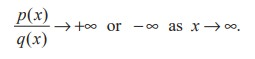Home | | Maths 11th std | Limits of rational functions

# Limits of rational functions

If R (x) = p(x)/ q (x) and the degree of the polynomial p(x) is greater than the degree of q(x), then

Limits of rational functions

If R (x) = p(x)/ q (x) and the degree of the polynomial p(x) is greater than the degree of q(x), thenIf the degree of q(x) is greater than the degree of p(x), thenFinally, if the degree of p(x) is equal to the degree of q(x), thenRemark

We reemphasize that statements such as f (x ) → ∞ as x → a , f (x ) → −∞ as x → a, and f (x ) → ∞ as x → ∞ , f (x ) → −∞ as x → ∞ mean that the limits do not exist. The symbol ∞ does not represent a number and should not be treated as a number.

Tags : Mathematics , 11th Mathematics : UNIT 9 : Differential Calculus Limits and Continuity
Study Material, Lecturing Notes, Assignment, Reference, Wiki description explanation, brief detail
11th Mathematics : UNIT 9 : Differential Calculus Limits and Continuity : Limits of rational functions | Mathematics